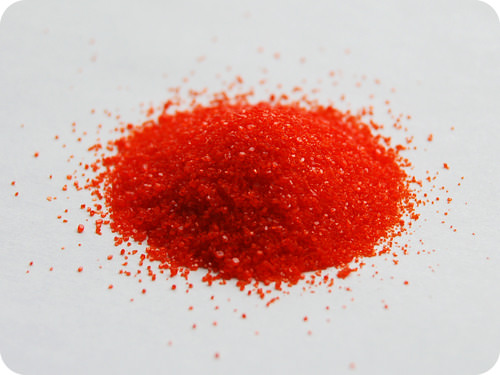# 5.22: Molar Mass

$$\newcommand{\vecs}{\overset { \rightharpoonup} {\mathbf{#1}} }$$ $$\newcommand{\vecd}{\overset{-\!-\!\rightharpoonup}{\vphantom{a}\smash {#1}}}$$$$\newcommand{\id}{\mathrm{id}}$$ $$\newcommand{\Span}{\mathrm{span}}$$ $$\newcommand{\kernel}{\mathrm{null}\,}$$ $$\newcommand{\range}{\mathrm{range}\,}$$ $$\newcommand{\RealPart}{\mathrm{Re}}$$ $$\newcommand{\ImaginaryPart}{\mathrm{Im}}$$ $$\newcommand{\Argument}{\mathrm{Arg}}$$ $$\newcommand{\norm}{\| #1 \|}$$ $$\newcommand{\inner}{\langle #1, #2 \rangle}$$ $$\newcommand{\Span}{\mathrm{span}}$$ $$\newcommand{\id}{\mathrm{id}}$$ $$\newcommand{\Span}{\mathrm{span}}$$ $$\newcommand{\kernel}{\mathrm{null}\,}$$ $$\newcommand{\range}{\mathrm{range}\,}$$ $$\newcommand{\RealPart}{\mathrm{Re}}$$ $$\newcommand{\ImaginaryPart}{\mathrm{Im}}$$ $$\newcommand{\Argument}{\mathrm{Arg}}$$ $$\newcommand{\norm}{\| #1 \|}$$ $$\newcommand{\inner}{\langle #1, #2 \rangle}$$ $$\newcommand{\Span}{\mathrm{span}}$$$$\newcommand{\AA}{\unicode[.8,0]{x212B}}$$Figure $$\PageIndex{1}$$ (Credit: Ben Mills (Wikimedia: Benjah-bmm27); Source: http://commons.wikimedia.org/wiki/File:Potassium-dichromate-sample.jpg(opens in new window); License: Public Domain)

### When creating a solution, how do I know how much of each substance to put in?

I want to make a solution that contains 1.8 moles of potassium dichromate. You do not have a balance calibrated in moles, but you do have one calibrated in grams. If you know the relationship between moles and the number of grams in a mole, you can use your balance to measure out the needed amount of material.

## Molar Mass

Molar mass is defined as the mass of one mole of representative particles of a substance. By looking at a periodic table, we can conclude that the molar mass of lithium is $$6.94 \: \text{g}$$, the molar mass of zinc is $$65.38 \: \text{g}$$, and the molar mass of gold is $$196.97 \: \text{g}$$. Each of these quantities contains $$6.02 \times 10^{23}$$ atoms of that particular element. The units for molar mass are grams per mole, or $$\text{g/mol}$$.

### Molar Masses of Compounds

The molecular formula of the compound carbon dioxide is $$\ce{CO_2}$$. One molecule of carbon dioxide consists of 1 atom of carbon and 2 atoms of oxygen. We can calculate the mass of one molecule of carbon dioxide by adding together the masses of 1 atom of carbon and 2 atoms of oxygen:

$12.01 \: \text{amu} + 2 \left( 16.00 \: \text{amu} \right) = 44.01 \: \text{amu}\nonumber$

The molecular mass of a compound is the mass of one molecule of that compound. The molecular mass of carbon dioxide is $$44.01 \: \text{amu}$$.

The molar mass of any compound is the mass in grams of one mole of that compound. One mole of carbon dioxide molecules has a mass of $$44.01 \: \text{g}$$, while one mole of sodium sulfide formula units has a mass of $$78.04 \: \text{g}$$. The molar masses are $$44.01 \: \text{g/mol}$$ and $$78.04 \: \text{g/mol}$$ respectively. In both cases, that is the mass of $$6.02 \times 10^{23}$$ representative particles. The representative particle of $$\ce{CO_2}$$ is the molecule, while for $$\ce{Na_2S}$$ it is the formula unit.

##### Example $$\PageIndex{1}$$: Molar Mass of a Compound

Calcium nitrate, $$\ce{Ca(NO_3)_2}$$, is used as a component in fertilizer. Determine the molar mass of calcium nitrate.

###### Solution

Step 1: List the known and unknown quantities and plan the problem.

###### Known
• Formula $$= \ce{Ca(NO_3)_2}$$
• Molar mass $$\ce{Ca} = 40.08 \: \text{g/mol}$$
• Molar mass $$\ce{N} = 14.01 \: \text{g/mol}$$
• Molar mass $$\ce{O} = 16.00 \: \text{g/mol}$$
###### Unknown
• molar mass Ca(NO3)2

First we need to analyze the formula. Since the $$\ce{Ca}$$ lacks a subscript, there is one $$\ce{Ca}$$ atom per formula unit. The 2 outside the parentheses means that there are two nitrate ions per formula unit and each nitrate ion consists of one nitrogen atom and three oxygen atoms per formula unit. Thus, $$1 \: \text{mol}$$ of calcium nitrate contains $$1 \: \text{mol}$$ of $$\ce{Ca}$$ atoms, $$2 \: \text{mol}$$ of $$\ce{N}$$ atoms, and $$6 \: \text{mol}$$ of $$\ce{O}$$ atoms.

###### Step 2: Calculate

Use the molar masses of each atom together with the number of atoms in the formula and add together.

$1 \: \text{mol} \: \ce{Ca} \times \frac{40.08 \: \text{g} \: \ce{Ca}}{1 \: \text{mol} \: \ce{Ca}} = 40.08 \: \text{g} \: \ce{Ca}\nonumber$

$2 \: \text{mol} \: \ce{N} \times \frac{14.01 \: \text{g} \: \ce{N}}{1 \: \text{mol} \: \ce{N}} = 28.02 \: \text{g} \: \ce{N}\nonumber$

$6 \: \text{mol} \: \ce{O} \times \frac{16.00 \: \text{g} \: \ce{O}}{1 \: \text{mol} \: \ce{O}} = 96.00 \: \text{g} \: \ce{O}\nonumber$

Molar mass of $$\ce{Ca(NO_3)_2} = 40.08 \: \text{g} + 28.02 \: \text{g} + 96.00 \: \text{g} = 164.10 \: \text{g/mol}$$

## Summary

• Calculations are described for the determination of molar mass of an atom or a compound.

## Review

1. What is the molar mass of Pb?
2. Where do you find the molar mass of an element?
3. How many moles of Cl are in one mole of the CaCl2?
4. How many moles of H are in one mole of the compound (NH4)3PO4?
5. Calculate the molar mass of CaCl2.

5.22: Molar Mass is shared under a CC BY-NC license and was authored, remixed, and/or curated by LibreTexts.